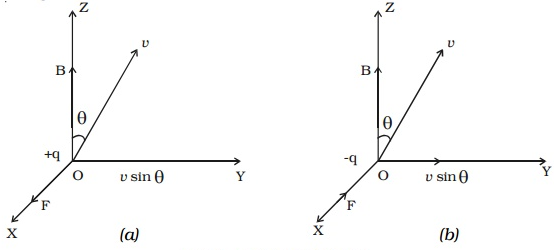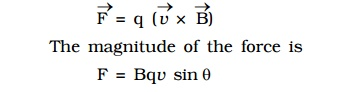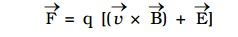Let us consider a uniform magnetic field of induction B acting along the Z-axis. A particle of charge + q moves with a velocity v in YZ plane making an angle θ with the direction of the field (Fig a). Under the influence of the field, the particle experiences a force F.H.A.Lorentz formulated the special features of the force F (Magnetic lorentz force) as under:

1. The force F on the charge is zero, if the charge is at rest. (i.e) the moving charges alone are affected by the magnetic field.

2. The force is zero, if the direction of motion of the charge is either parallel or anti-parallel to the field and the force is maximum, when the charge moves perpendicular to the field.

3. The force is proportional to the magnitude of the charge (q)

4. The force is proportional to the magnetic induction (B)

5. The force is proportional to the speed of the charge (v)

6. The direction of the force is oppositely directed for charges of opposite sign (Fig b).

All these results are combined in a single expression asSince the force always acts perpendicular to the direction of motion of the charge, the force does not do any work.

In the presence of an electric field E and magnetic field B, the total force on a moving charged particle is